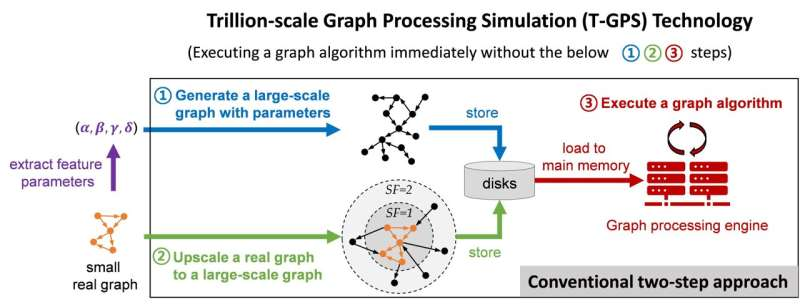# T-GPS processes a graph with a trillion edges

A KAIST research team has developed a new technology that enables the processing of a large-scale graph algorithm without storing the graph in the main memory or on disk. Named as T-GPS (Trillion-scale Graph Processing Simulation) by the developer Professor Min-Soo Kim from the School of Computing at KAIST, it can process a graph with one trillion edges using a single computer.

Graphs are widely used to represent and analyze real-world objects in many domains such as social networks, business intelligence, biology, and neuroscience. As the number of graph applications increases rapidly, developing and testing new graph algorithms is becoming more important than ever before. Nowadays, many industrial applications require a graph algorithm to process a large-scale graph (e.g., one trillion edges). So, when developing and testing graph algorithms such for a large-scale graph, a synthetic graph is usually used instead of a real graph. This is because sharing and utilizing large-scale real graphs is very limited due to their being proprietary or being practically impossible to collect.Conventionally, developing and testing graph algorithms is done via the following two-step approach: generating and storing a graph and executing an algorithm on the graph using a graph processing engine. The first step generates a synthetic graph and stores it on disks. The synthetic graph is usually generated by either parameter-based generation methods or graph upscaling methods. The former extracts a small number of parameters that can capture some properties of a given real graph and generates the synthetic graph with the parameters. The latter upscales a given real graph to a larger one so as to preserve the properties of the original real graph as much as possible.

The second step loads the stored graph into the main memory of the graph processing engine such as Apache GraphX and executes a given graph algorithm on the engine. Since the size of the graph is too large to fit in the main memory of a single computer, the graph engine typically runs on a cluster of several tens or hundreds of computers. Therefore, the cost of the conventional two-step approach is very high.

The research team solved the problem of the conventional two-step approach. It does not generate and store a large-scale synthetic graph. Instead, it just loads the initial small real graph into main memory. Then, T-GPS processes a graph algorithm on the small real graph as if the large-scale synthetic graph that should be generated from the real graph exists in main memory. After the algorithm is done, T-GPS returns the exactly same result as the conventional two-step approach.

The research team showed that T-GPS can process a graph of 1 trillion edges using a single computer, while the conventional two-step approach can only process of a graph of 1 billion edges using a cluster of eleven computers of the same specification. Thus, T-GPS outperforms the conventional approach by 10,000 times in terms of computing resources. The team also showed that the speed of processing an algorithm in T-GPS is up to 43 times faster than the conventional approach. This is because T-GPS has no network communication overhead, while the conventional approach has a lot of communication overhead among computers.

https://techxplore.com/news/2021-05-t-gps-graph-trillion-edges.html